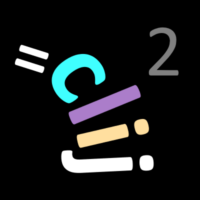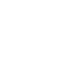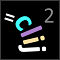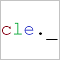# CLIJ2GPU accelerated image processing for everyone

CLIJ2 home

## neighborsOfNeighborsDetermines neighbors of neigbors from touch matrix and saves the result as a new touch matrix.

Categories: Filter, Graphs

Availability: Available in Fiji by activating the update sites clij and clij2. This function is part of clij2_-2.5.0.1.jar.

### Usage in ImageJ macro

``````Ext.CLIJ2_neighborsOfNeighbors(Image touch_matrix, Image neighbor_matrix_destination);
``````

### Usage in object oriented programming languages

Java
```// init CLIJ and GPU
import net.haesleinhuepf.clij2.CLIJ2;
import net.haesleinhuepf.clij.clearcl.ClearCLBuffer;
CLIJ2 clij2 = CLIJ2.getInstance();

// get input parameters
ClearCLBuffer touch_matrix = clij2.push(touch_matrixImagePlus);
neighbor_matrix_destination = clij2.create(touch_matrix);
```
```// Execute operation on GPU
clij2.neighborsOfNeighbors(touch_matrix, neighbor_matrix_destination);
```
```// show result
neighbor_matrix_destinationImagePlus = clij2.pull(neighbor_matrix_destination);
neighbor_matrix_destinationImagePlus.show();

// cleanup memory on GPU
clij2.release(touch_matrix);
clij2.release(neighbor_matrix_destination);
```
Matlab
```% init CLIJ and GPU
clij2 = init_clatlab();

% get input parameters
touch_matrix = clij2.pushMat(touch_matrix_matrix);
neighbor_matrix_destination = clij2.create(touch_matrix);
```
```% Execute operation on GPU
clij2.neighborsOfNeighbors(touch_matrix, neighbor_matrix_destination);
```
```% show result
neighbor_matrix_destination = clij2.pullMat(neighbor_matrix_destination)

% cleanup memory on GPU
clij2.release(touch_matrix);
clij2.release(neighbor_matrix_destination);
```
Icy JavaScript
```// init CLIJ and GPU
importClass(net.haesleinhuepf.clicy.CLICY);
importClass(Packages.icy.main.Icy);

clij2 = CLICY.getInstance();

// get input parameters
touch_matrix_sequence = getSequence();
touch_matrix = clij2.pushSequence(touch_matrix_sequence);
neighbor_matrix_destination = clij2.create(touch_matrix);
```
```// Execute operation on GPU
clij2.neighborsOfNeighbors(touch_matrix, neighbor_matrix_destination);
```
```// show result
neighbor_matrix_destination_sequence = clij2.pullSequence(neighbor_matrix_destination)
// cleanup memory on GPU
clij2.release(touch_matrix);
clij2.release(neighbor_matrix_destination);
```
clEsperanto Python (experimental)
```import pyclesperanto_prototype as cle

cle.neighbors_of_neighbors(touch_matrix, neighbor_matrix_destination)

```

Imprint